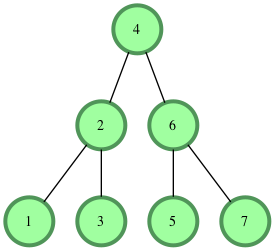## Algorithm

Problem Name: Data Structures - Tree: Height of a Binary Tree

In this HackerRank in Data Structures - Tree: Height of a Binary Tree solutions

The height of a binary tree is the number of edges between the tree's root and its furthest leaf. For example, the following binary tree is of height 2:Function Description

Complete the getHeight or height function in the editor. It must return the height of a binary tree as an integer.

getHeight or height has the following parameter(s):

• root: a reference to the root of a binary tree.

Note -The Height of binary tree with single node is taken as zero.

Input Format

The first line contains an integer n, the number of nodes in the tree.
Next line contains n space separated integer where

th integer denotes node[i].data.

Note: Node values are inserted into a binary search tree before a reference to the tree's root node is passed to your function. In a binary search tree, all nodes on the left branch of a node are less than the node value. All values on the right branch are greater than the node value.

Constraints

1 <= node.data[i] <= 20

1 <= n <= 20

Output Format

Your function should return a single integer denoting the height of the binary tree.

Sample InputSample Output

3


Explanation

The longest root-to-leaf path is shown below:There are 4 nodes in this path that are connected by 3 edges, meaning our binary tree's height = 3

## Code Examples

### #1 Code Example with C Programming

Code - C Programming


#include <stdio.h>
#include <string.h>
#include <math.h>
#include <stdlib.h>

struct node {

int data;
struct node *left;
struct node *right;

};

struct node* insert( struct node* root, int data ) {

if(root == NULL) {

struct node* node = (struct node*)malloc(sizeof(struct node));

node->data = data;

node->left = NULL;
node->right = NULL;
return node;

} else {

struct node* cur;

if(data <= root->data) {
cur = insert(root->left, data);
root->left = cur;
} else {
cur = insert(root->right, data);
root->right = cur;
}

return root;
}
}

/* you only have to complete the function given below.
node is defined as

struct node {

int data;
struct node *left;
struct node *right;

};

*/
int getHeight(struct node* root)
{

int counter_right = 0;
int counter_left = 0;

if (root == NULL)
{
return -1;
}

counter_left = getHeight(root->left);
counter_left++;

counter_right = getHeight(root->right);
counter_right++;

if (counter_left > counter_right)
{
return counter_left;
}

return counter_right;
}

int main() {

struct node* root = NULL;

int t;
int data;

scanf("%d", &t);

while(t-- > 0) {
scanf("%d", &data);
root = insert(root, data);
}

printf("%d",getHeight(root));
return 0;
}

Copy The Code &

### #2 Code Example with C++ Programming

Code - C++ Programming


#include <bits/stdc++.h>

using namespace std;

class Node {
public:
int data;
Node *left;
Node *right;
Node(int d) {
data = d;
left = NULL;
right = NULL;
}
};

class Solution {
public:
Node* insert(Node* root, int data) {
if(root == NULL) {
return new Node(data);
} else {
Node* cur;
if(data <= root->data) {
cur = insert(root->left, data);
root->left = cur;
} else {
cur = insert(root->right, data);
root->right = cur;
}

return root;
}
}
/*The tree node has data, left child and right child
class Node {
int data;
Node* left;
Node* right;
};

*/
int height(Node* root) {
int leftHeight=-1,rightHeight=-1;
if(root->left){
leftHeight=height(root->left);
}
if(root->right)
rightHeight=height(root->right);
return max(leftHeight,rightHeight)+1;
}

}; //End of Solution

int main() {

Solution myTree;
Node* root = NULL;

int t;
int data;

std::cin >> t;

while(t-- > 0) {
std::cin >> data;
root = myTree.insert(root, data);
}

int height = myTree.height(root);

std::cout << height;

return 0;
}

Copy The Code &

### #3 Code Example with Java Programming

Code - Java Programming


import java.util.*;
import java.io.*;

class Node {
Node left;
Node right;
int data;

Node(int data) {
this.data = data;
left = null;
right = null;
}
}

class Solution {

/*
class Node
int data;
Node left;
Node right;
*/
public static int height(Node root) {
if(root == null) {
return -1;
}
return Math.max(height(root.left), height(root.right)) + 1;
}

public static Node insert(Node root, int data) {
if(root == null) {
return new Node(data);
} else {
Node cur;
if(data <= root.data) {
cur = insert(root.left, data);
root.left = cur;
} else {
cur = insert(root.right, data);
root.right = cur;
}
return root;
}
}

public static void main(String[] args) {
Scanner scan = new Scanner(System.in);
int t = scan.nextInt();
Node root = null;
while(t-- > 0) {
int data = scan.nextInt();
root = insert(root, data);
}
scan.close();
int height = height(root);
System.out.println(height);
}
}

Copy The Code &

### #4 Code Example with Python Programming

Code - Python Programming


class Node:
def __init__(self, info):
self.info = info
self.left = None
self.right = None
self.level = None

def __str__(self):
return str(self.info)

class BinarySearchTree:
def __init__(self):
self.root = None

def create(self, val):
if self.root == None:
self.root = Node(val)
else:
current = self.root

while True:
if val < current.info:
if current.left:
current = current.left
else:
current.left = Node(val)
break
elif val > current.info:
if current.right:
current = current.right
else:
current.right = Node(val)
break
else:
break

# Enter your code here. Read input from STDIN. Print output to STDOUT
'''
class Node:
def __init__(self,info):
self.info = info
self.left = None
self.right = None

// this is a node of the tree , which contains info as data, left , right
'''
def height(root):
leftHeight = 0
rightHeight = 0

if(root.left):
leftHeight = height(root.left) + 1

if(root.right):
rightHeight = height(root.right) + 1

if(leftHeight > rightHeight):
return leftHeight
else:
return rightHeight

tree = BinarySearchTree()
t = int(input())

arr = list(map(int, input().split()))

for i in range(t):
tree.create(arr[i])

print(height(tree.root))

Copy The Code &